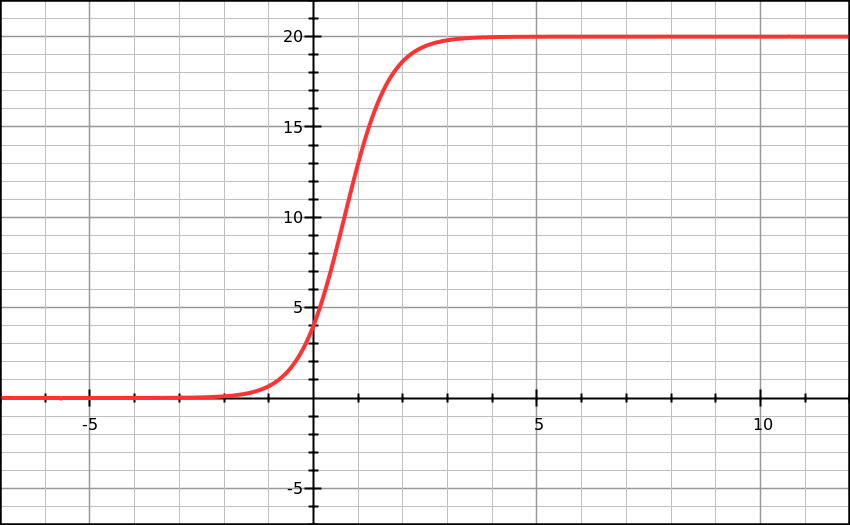# The Logistic Function (Population Growth)

Calculus Level 3Exponential functions are prevalent in many problems in science, business, economics, medicine, and sociology. One important function, the logistic function, is often used to analyze population growth that is limited by natural environmental factors. The general form of the logistic function is

$P(t)=\frac{A}{1+Be^{-Ct}},$ where constants $A$, $B$, and $C$ are usually determined experimentally.

Suppose that population in a town is modeled by

$P(t)=\frac{20,000}{1+4e^{-2t}},$ where $P(t)$ is number of population and $t$ is time in year. Let $t_1$ be the time when the population growth rate begin to decline and $t_2$ be the time when the population reach $80$ percent of its limit, then $t_1+t_2$ can be expressed as $T\ln 2$. Determine the value of $T$.

×

Problem Loading...

Note Loading...

Set Loading...